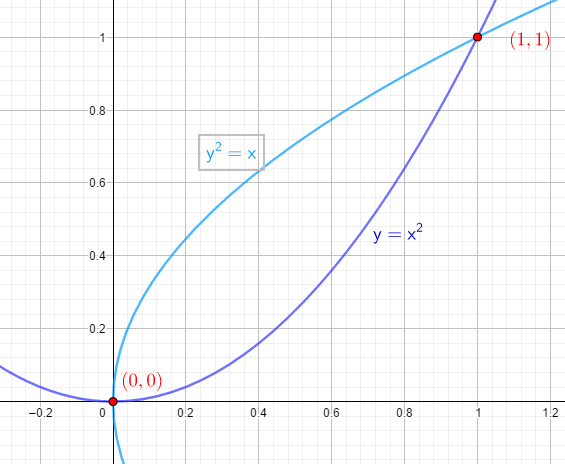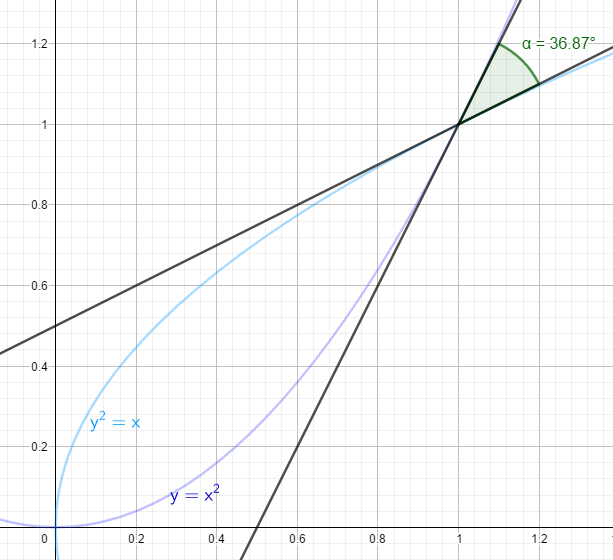### Math Notes

Subjects

#### Differential Calculus Solutions

##### Topics || Problems

Find the angle between parabolas $$y^2 =x$$ and $$y =x^2$$ at the points of their intersections.

Find the intersection:

$$y^2 = \sqrt{y}$$

$$y^4 = y$$

$$y^4 - y = 0$$

$$y=0 ~ and ~ y=1$$

Intersection $$(0,0), (1,1)$$At $$(0,0)$$

Slope$$(m_1)$$ of $$y^2 =x$$.

$$m_1 = \frac{dy}{dx} = \frac{1}{2y}$$

$$m_1 = \frac{dy}{dx} = \frac{1}{0}$$

$$m_1 = \frac{dy}{dx} = ~undefined$$, the tangent line is a horizontal line.

Slope$$(m_2)$$ of $$y =x^2$$.

$$m_2 = \frac{dy}{dx} = 2x$$

$$m_2 = \frac{dy}{dx} = 0$$ a vertical line

The angle between line is $$90^o$$.

At $$(1,1)$$

Slope$$(m_1)$$ of $$y^2 =x$$.

$$m_1 = \frac{dy}{dx} = \frac{1}{2y}$$

$$m_1 = \frac{dy}{dx} = \frac{1}{2}$$

Slope$$(m_2)$$ of $$y =x^2$$.

$$m_2 = \frac{dy}{dx} = 2x$$

$$m_2 = \frac{dy}{dx} = 2(1)$$

$$m_2 = \frac{dy}{dx} = 2$$

The angle between line is $$\tan \theta= \frac{m_2 - m_1}{1+ m_2m_1}$$.

$$\theta = ~arctan (\frac{2-0.5}{1+2(0.5)})$$

$$\theta = 36.87^o$$